TrigonometryShowing results 11 to 20 of 36, on page 2 of 4 Arbitrary Triangle Trigonometric Definitions Trigonometric Identities Trigonometric Properties
 DESCRIPTION EQUATION Half Angle Identity - Cosine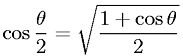Half Angle Identity - Sine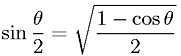Law of Cosines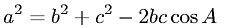Law of SinesOdd Symmetry Property - Cosecant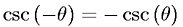Odd Symmetry Property - Sine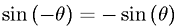Odd Symmetry Property - Tangent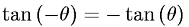Pythagorean Property - Cotangent and CosecantPythagorean Property - Sine and Cosine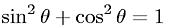Pythagorean Property - Tangent and Secant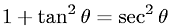Go to page: 1  2  3  4